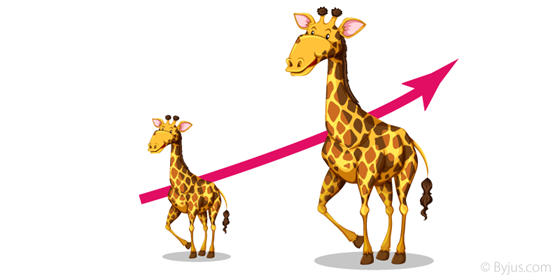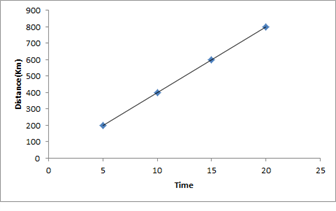# Direct Proportion

Direct proportion or direct variation is the relation between two quantities where the ratio of the two is equal to a constant value. It is represented by the proportional symbol, ∝. In fact, the same symbol is used to represent inversely proportional, the matter of the fact that the other quantity is inverted here.

For example, x and y are two quantities or variables which are linked with each other directly, then we can say x ∝ y. When we remove the proportionality symbol, the ratio of x and y becomes equal to a constant, such as x/y = C, where C is a constant. But in the case of inverse proportion, x and y are denoted as x ∝ 1/y or xy = C.

## Direct proportion Examples in Real Life

In our day-to-day life, we observe that the variations in the values of various quantities depending upon the variation in values of some other quantities.For example: if the number of individuals visiting a restaurant increases, earning of the restaurant also increases and vice versa. If more number of people are employed for the same job, the time taken to accomplish the job decreases.

Sometimes, we observe that the variation in the value of one quantity is similar to the variation in the value of another quantity that is when the value of one quantity increases then the value of other quantity also increases in the same proportion and vice versa. In such situations, two quantities are termed to exist in direct proportion.Some more examples are:

• Speed is directly proportional to distance.
• The cost of the fruits or vegetable increases as the weight for the same increases.

### Direct Proportion Symbol and Constant of Proportionality

The symbol for “direct proportional” is ‘∝’  (One should not confuse with the symbol for infinity ∞). Two quantities existing in direct proportion can be expressed as;

x ∝ y

x/y = k

x = ky

k is a non-zero constant of proportionality.

Where x and y are the value of two quantities and k are a constant known as the constant of proportionality. If x1, y1 is the initial values and x2, y2 are the final values of quantities existing in direct proportion. They can be expressed as,

$$\begin{array}{l}\frac{x_1}{y_1} = \frac{x_2}{y_2}\end{array}$$

Example: a machine manufactures 20units per hour

The units that machine manufactures is directly proportional to how many hours it has worked.

More works the machine does, more are the units manufactured; in direct proportion.

This could be written as:

Units  Hours Worked

• If it works 2 hours we get 40 Units
• If it works 4 hours we get 80 Units

### Direct proportion Questions and Answers

Q.1: An electric pole, 7 meters high, casts a shadow of 5 meters. Find the height of a tree that casts a shadow of 10 meters under similar conditions.

Solution: Let the height of the tree be x meters. We know that if the height of the pole increases the length of shadow will also increase in same proportion. Hence, we observe that the height of the tree and the length of its shadow exist in direct proportion. In other words height of pole is directly proportional to the length of its shadow. Thus,

$$\begin{array}{l}\frac{x_1}{y_1} = \frac{x_2}{y_2}\end{array}$$
$$\begin{array}{l}\frac{7}{5} =\frac{x}{10}\end{array}$$

x = 14 meters

Q.2: A train travels 200 km in 5 hours. How much time it will take to cover 600 km?

Solution: Let the time taken be T hours. We know that time taken is directly proportional to distance covered. Hence,

$$\begin{array}{l}\frac{x_1}{y_1} = \frac{x_2}{y_2}\end{array}$$
$$\begin{array}{l}\Rightarrow~\frac{200}{5} = \frac{600}{T}\end{array}$$

T = 15 hoursQ. 3: The scale of a map is given as 1:20000000. Two cities are 4cm apart on the map. Find the actual distance between them.

Solution: Map distance is 4 cm.

Let the actual distance be x cm, then 1:20000000 = 4:x.

1/20000000 = 4/x

⇒ x = 80000000 cm = 800 km

For a detailed discussion on the concept of direct proportion and developing a relationship between two quantities based on direct proportion, inverse proportion and other topics download BYJU’S – The Learning App from Google Play Store and watch interactive videos. Also, take free tests to practice for exams.

Test your knowledge on Direct Proportion